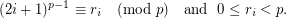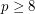# Alexa's Conjecture on Primality

 Importance: Medium ✭✭
 Author(s): Alexa
 Subject: Number Theory
 Keywords: primality
 Posted by: princeps on: March 28th, 2012
Definition   Letbe the unique integer (with respect to a fixed) such thatConjecture   A natural numberis a prime iffThe conjecture is obviously true whenis prime, so it suffices to check whenis composite.

## Bibliography

* indicates original appearance(s) of problem.

### counter-example for p=66

formula works for p from 8 through 100, except for p=66.

### counter-example for p=66

Thanks , I have corrected statement .

### don't work either

your new statement is ambiguous (which r_i should one choose inside the sum?). I'm assuming you're just trying to move the "mod p" to apply to the sum only (and not to the RHS). If that's what you're doing, it still doesn't work. Same counter-examples at p=66, 102, 246 and 492 for p from 8 to 500.

### donit work either

Yes it works .I have checked statement up to 10^6 ,there is no counterexample...

### still ambiguous

then please re-word your conjecture, because as it stands, it's ambiguous and not true. It's ambiguous, because the way you defined r_i, one could have chosen r_i, r_i + p, r_i + 2p etc., but when you plug these into the sum, you get a different sum and the equality doesn't make sense.

### still not good

your modified version now reduces back to putting mod p on the LHS of the equation, which as I've pointed out above, doesn't work (see counter-examples I gave). Where did you come up with this conjecture? Is there any published reference for it?

### Fixed

I've corrected the statement on Alexa's behalf. This version holds up to at least 100 million.